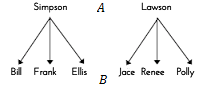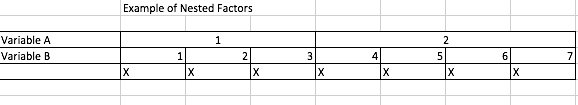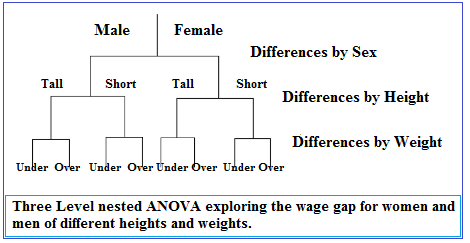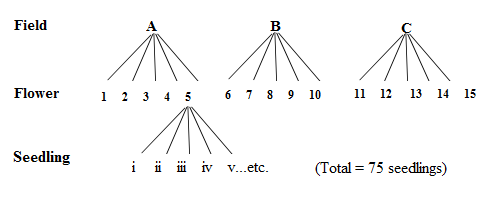# Nested Model, ANOVA and Factors: Simple Definitions and Examples

Share on

ANOVA > Nested Model

Contents:

## What is a Nested Model?

Very simply, “nested” means that one model is a subset of another. For example, take a model for pregnancy outcomes that includes four categorical independent variables:

• Age,
• Weight,
• Pre-existing conditions,
• Hereditary factors.

Several smaller models can be derived from this main one, and each is “nested” inside the main model. For example:

1. Age and weight,
2. Weight and pre-existing conditions,
3. Age and hereditary factors.

Basically, if you can get one model by constraining parameters of another, those models are nested. For example, the set of normal distribution models contains an infinite number of nested models, including normal distributions with means of 0, 1, or 99.

## Uses

Nested models are used for several statistical tests and analyses, including multiple regression, likelihood-ratio tests, conjoint analysis, and independent of irrelevant alternatives (IIA). While the above definition should give you a general sense of what a nested model is, the definition gets a bit more technical depending on where you are using is. For example:

In multiple regression and structural equation modeling (SEM), the idea is the same — that one model is nested inside another. More technically, both models must have identical terms and one of the models must have one or more extra terms. For example:

• y = β0 + β1x1 + β2x2 + 10
• y = β0 + β1x1 + β2x2 + β3x1x2 + 10

The larger model is called the full model and the smaller model is called the reduced model.

Caution: not all nested models are as obvious as the ones I have highlighted above. Rigdon (1999) suggest caution when deciding to analyze nested models because of this fact. At the time of writing there isn’t any software that can analyze if two different structural models are similar (Bentler & Satorra).

## Nested Factors

Nested factors ‘fit inside each other’.

As a reminder, a “factor” is a set of observed variables that have similar response patterns. Two factors A and B are nested if there is an entirely different set of values of B for every value of A.As an example, let’s say factor “A” is family and factor “B” is children. A child can be Simpson or Lawson, but not both. Bill, Frank, or Ellis are Simpson; Jace, Renee, or Polly are Lawson. These two factors (family/child) are nested because any given child exists in only one family. In more formal terms, we say that every value of B exists for one and only one value of A.

## Examples of Nested Factors.

Imagine a product tester needs to test lead and arsenic levels in canned baked beans produced by a certain well-known brand. He might visit three different factories, test two different batches in each factory, and open five cans per batch. Factory, Batch #, and Can ID# are three different variables, but they are nested. Every can exists only in one particular batch, and each batch exists in only one factory.

Or suppose a class of children was surveyed on their favorite snack which was either sweet or salty (factor A). Some said they preferred savory snacks, others said they liked sweet snacks better. Among the children who liked savory snacks were specific brands (factor B). Some children preferred Cheez-its(1), nachos(2), spicy popcorn(3) or Slim Jims(4). Other children preferred sweet snacks: ice cream(1), chocolate(2), fruit(3) and candy(4).

The two factors A and B are nested. Each of the snack variables is included in exactly one of the sweet/savory variable distinctions.

## Notation for Nested Factors

The subscript j(i) indicates that the factor indexed by j is nested in the factor indexed by i. In the above snack example, you could index A with i = 1, 2 and B with j = 1, 2, 3 ,4. Note that even though the indexing of B (brand) is repeated across the two instances of A (sweet or salty), the actual values of B are different for both. For example, 1(2) (preference for nachos) is not the same as 2(2) (preference for chocolate).

## Determining if Factors are Nested

Sometimes it isn’t immediately obvious whether or not factors are nested. The easiest way to check is to make a table; if  every value of B is nonzero for only one value of A, B is nested in A.In the table above, numbers were randomly assigned to each value of each variable. Columns with no data (zero everywhere) were deleted; For example, all instances of B = 5 are in A = 2. The column A = 1, B = 5 is empty, so is not included in the table.

## What is Nested ANOVA?

A nested ANOVA (also called a hierarchical ANOVA) is an extension of a simple ANOVA for experiments where each group is divided into two or more random subgroups. It tests to see if there is variation between groups, or within nested subgroups of the attribute variable. You should use nested ANOVA when you have:

## Examples

Let’s say you wanted to investigate the wage gap between men and women. You also think that height affects wages (which is true — see The Atlantic’s story on Why Tall People Make More) as does obesity (also true: see Forbes’ story The Price of Obesity). Your factors or levels (sex, height, weight) are nested within each other. For example, “weight” is not a standalone factor — it’s nested under male/female. The following image shows the hierarchical model:In the following example, 5 different seedlings have been sampled from 5 different flowers in two different fields A and B:## Model I and Model II in Nested ANOVA

A model I ANOVA (also called a fixed-effects model) is where the treatments are fixed by the experimenter. For example, if you are comparing how different weights affect health you might choose specific weight ranges. If a nested ANOVA has a highest level of Model I, it’s called a mixed model nested ANOVA.

Model II ANOVAs are where the treatments are random and not fixed. For example, instead of the researcher choosing weights, they would be chosen at random. If a nested ANOVA has a highest level of model II, it’s called a pure model II nested ANOVA.

## Nested vs. Crossed Designs

While nested models can be represented by a purely hierarchical graph — such as the ones above — crossed models involve some crossover between the levels of independent variable. An example of a pure crossed model is where two groups of students are taught different ways to solve math problems by teacher A and teacher B. As all students in both groups are exposed to teacher A’s methods and teacher B’s methods, the model is crossed. If it was nested, one group of students would only experience one teacher’s methods.

Crossed designs are preferable, because they are better at detecting differences between groups than nested models. However, it may not be possible to always use crossed models — some experiments necessitate the used of nested models.

In many experiments, it may not be clear if your model is nested or crossed — and in some cases you might have a combination of both. Figuring out if your design is nested or not can be challenging. Drawing a hierarchical graph like the ones above can help.

The above example of the wage gap between men and women would be crossed instead of nested if it was possible for the factor levels to cross over. For example, if a man could be both short and tall, or normal weight and overweight. While this is theoretically possible (for example, you could have twins, one of whom is overweight and one is normal weight), in this case the scenario is not crossed.

## References

Bentler, P. & Satorra, A. (2010). Testing Model Nesting and Equivalence. Psychol Methods. 2010 Jun; 15(2): 111–123. Retrieved 9/19/2016 from http://www.ncbi.nlm.nih.gov/pmc/articles/PMC2929578/.
Carriquiry, A. Multiple Regression. Retrieved 9/19/2016 from: http://www.public.iastate.edu/~alicia/stat328/Multiple%20regression%20-%20nested%20models.pdf
Doncaster & Davey (2007). Analysis of Variance and Covariance: How to Choose and Construct Models for the Life Sciences. Cambridge University Press.
Purdue Statistics. A Look at Nested Factors. Retrieved September 17, 2017 from: http://www.stat.purdue.edu/~bacraig/notes1/topic19.pdf
Rigdon, E.E. (1999). Using the Friedman method of ranks for model comparison in structural equation modeling. Structural equation modeling, 6(3), 219-232

CITE THIS AS:
Stephanie Glen. "Nested Model, ANOVA and Factors: Simple Definitions and Examples" From StatisticsHowTo.com: Elementary Statistics for the rest of us! https://www.statisticshowto.com/nested-model-anova-factors/
---------------------------------------------------------------------------Need help with a homework or test question? With Chegg Study, you can get step-by-step solutions to your questions from an expert in the field. Your first 30 minutes with a Chegg tutor is free!